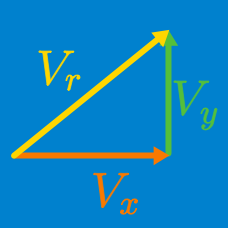Classical Mechanics

# Different Representations of Vectors

A two-dimensional vector's initial point is $(3,-2)$ and the vector has a magnitude of $12.$ This vector forms a $45^{\circ}$ angle with the $+x$ direction. What is the terminal point of the vector?

A woman moves $4\text{ m}$ east, $1\text{ m}$ south, and $4\text{ m}$ west. What is the direction of the displacement vector of the woman?

An object on a tabletop moves in a straight line, in a direction that forms a $60^{\circ}$ angle with a ruler. The position of the object read from the ruler was $10\text{ cm}$ when it started moving. The object then stops when its position read from the ruler is $32\text{ cm}.$ What is the displacement of the object?

A vector's initial point is $(4,8)$ and terminal point is $(16,-8)$ on the coordinate plane. Find the magnitude of the vector.

Tom walked $21\text{ miles}$ north, and then $28\text{ miles}$ east. How far is he from his original location?

×